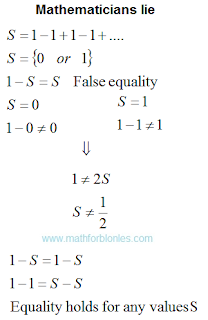## 10/19/2019

### Mathematicians lie

I watched the video One minus one plus one minus one - Numberphile. Mathematicians lie. They did not perform an equality test in the course of their reasoning.Mathematicians lie

Confirmation of my reasoning about infinite sets.

If you take a closer look at all the manipulations, the error will immediately become visible. The example is written on one line and "equality" is based on the optical illusion of similarity between two different mathematical expressions. Let's write down all actions in a column, starting with the equality 1-S = S.False equality

As you can see from this example, on opposite sides of the equal sign there are a different number of terms, on the left there is one more term. Equality cannot be fulfilled if this term is not equal to zero. So the given "proof" is a common lie. I have no doubt that other "proofs" of the sum of this infinite series are also based on false statements.